Next: Evaluation of type B Up: Approximate methods Previous: Linearization   Contents

## BIPM and ISO recommendations

In this section we compare the results obtained in the previous section with the recommendations of the Bureau International des Poids et Mesures (BIPM) and the International Organization for Standardization (ISO) on the expression of experimental uncertainty''.
1. The uncertainty in the result of a measurement generally consists of several components which may be grouped into two categories according to the way in which their numerical value is estimated:
A:
those which are evaluated by statistical methods;
B:
those which are evaluated by other means.

There is not always a simple correspondence between the classification into categories A or B and the previously used classification into random'' and systematic'' uncertainties. The term systematic uncertainty'' can be misleading and should be avoided.

The detailed report of the uncertainty should consist of a complete list of the components, specifying for each the method used to obtain its numerical result.
Essentially the first recommendation states that all uncertainties can be treated probabilistically. The distinction between types A and B is subtle and can be misleading if one thinks of statistical methods'' as synonymous with probabilistic methods'', as is currently the case in High Energy Physics. Here statistical'' has the classical meaning of repeated measurements.

2. The components in category A are characterized by the estimated variances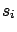(or the estimated standard deviations''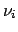) and the number of degrees of freedom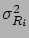. Where appropriate, the covariances should be given.
The estimated variances correspond to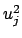of the previous section. The degrees of freedom are related to small samples and to the Student t distribution. The problem of small samples is not discussed in these notes, but clearly this recommendation is a relic of frequentistic methods. With the approach followed in these notes there is no need to talk about degrees of freedom, since the Bayesian inference defines the final probability function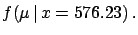completely6.2.

3. The components in category B should be characterized by quantities, which may be considered as approximations to the corresponding variances, the existence of which is assumed. The quantitiesmay be treated like variances and the quantities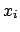like standard deviations. Where appropriate, the covariances should be treated in a similar way.
Clearly, this recommendation is meaningful only in a Bayesian framework.

4. The combined uncertainty should be characterized by the numerical value obtained by applying the usual method for the combination of variances. The combined uncertainty and its components should be expressed in the form of standard deviations''.
This is what we have found in () and ().
5. If, for particular applications, it is necessary to multiply the combined uncertainty by a factor to obtain an overall uncertainty, the multiplying factor used must always be stated.
This last recommendation states once more that the uncertainty is by default'' the standard deviation of the true value distribution. Any other quantity calculated to obtain a credibility interval with a certain probability level should be clearly stated.
To summarize, these are the basic ingredients of the BIPM/ISO recommendations.
subjective definition of probability:
it allows variances to be assigned conceptually to any physical quantity which has an uncertain value;
uncertainty as standard deviation
combined standard uncertainty:
it is obtained by the usual formula of error propagation'' and it makes use of variances, covariances and first derivatives;
central limit theorem:
it makes, under proper conditions, the true value normally distributed if one has several sources of uncertainty.

Consultation of the Guide is recommended for further explanations about the justification of the standards, for the description of evaluation procedures, and for examples. I would just like to end this section with some examples of the evaluation of type B uncertainties and with some words of caution concerning the use of approximations and of linearization.Next: Evaluation of type B Up: Approximate methods Previous: Linearization   Contents
Giulio D'Agostini 2003-05-15